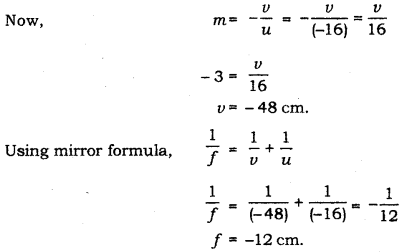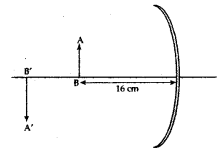# List the sign conventions for reflection of light by spherical mirrors

List the sign conventions for reflection of light by spherical mirrors. Draw a diagram and apply these conventions in the determination of focal length of a spherical mirror which forms a three times magnified real image of an object placed 16 cm in front of it.

Sign conventions for spherical mirrors:
I. Objects are always placed to the left of the mirror i.e. light must fall on the mirror from left to right.

II. All distances are measured from the pole of the mirror.

III. Distances along the direction of the incident ray ( along positive X - axis) are taken as positive, while distances along the direction of the reflected ray (along negative X-axis) are taken as negative.

IV. Heights measured perpendicular to and above the principal axis (along positive Y-axis) are taken as positive.

V. Heights measured perpendicular to and below the principal axis (along negative Y-axis) are taken as negative.Given,
m = -3 (Real image is inverted always)
u = -16 cm